# How to Calculate and Solve for Independent Events | Probability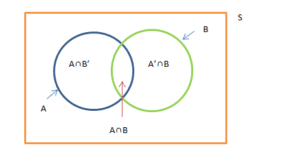The image above represents independent events.

To compute for independent events, four essential parameters are needed and these parameters are Number of Times Event A can occur (xA), Number of Times Event B can occur (xB) and Total Number of All Possible Outcomes (N).

The formula for calculating independent events:

P(A and B) = P(A) x P(B)

Where;

P(A and B) = Independent events
xA = Number of Times Event A can occur
xB = Number of Times Event B can occur
N = Total Number of All Possible Outcomes
P(A) = xAN
P(B) = xBN

Let’s solve an example;
Find the independent events when the number of times event A can occur is 11, number of times event B can occur is 15 and the total number of all possible outcomes is 22.

This implies that;

xA = Number of Times Event A can occur = 11
xB = Number of Times Event B can occur = 15
N = Total Number of All Possible Outcomes = 22

P(A and B) = P(A) x P(B)
P(A and B) = xAN x xBN
P(A and B) = 1122 x 1522
P(A and B) = (11)(15)(22)(22)
P(A and B) = 165484
Dividing the numerator and denominator by 11
P(A and B) = 1544
P(A and B) = 0.3409

Therefore, the independent events is 0.3409.

Nickzom Calculator – The Calculator Encyclopedia is capable of calculating the independent events.

To get the answer and workings of the independent events using the Nickzom Calculator – The Calculator Encyclopedia. First, you need to obtain the app.

You can get this app via any of these means:

To get access to the professional version via web, you need to register and subscribe for NGN 1,500 per annum to have utter access to all functionalities.
You can also try the demo version via https://www.nickzom.org/calculator

Apple (Paid) – https://itunes.apple.com/us/app/nickzom-calculator/id1331162702?mt=8
Once, you have obtained the calculator encyclopedia app, proceed to the Calculator Map, then click on Probability under Mathematics.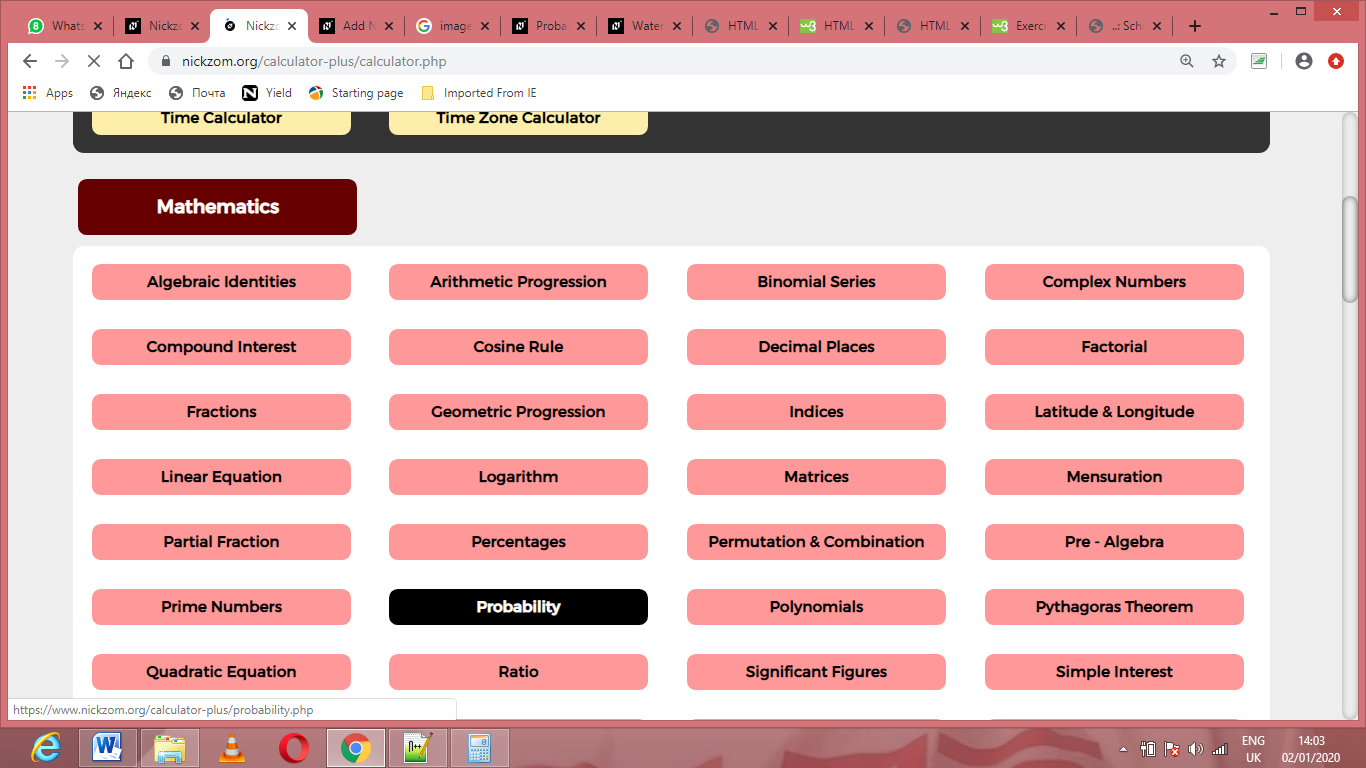Now, Click on Independent Events under ProbabilityThe screenshot below displays the page or activity to enter your values, to get the answer for the independent events according to the respective parameters which are the Number of Times Event A can occur (xA), Number of Times Event B can occur (xB) and Total Number of All Possible Outcomes (N).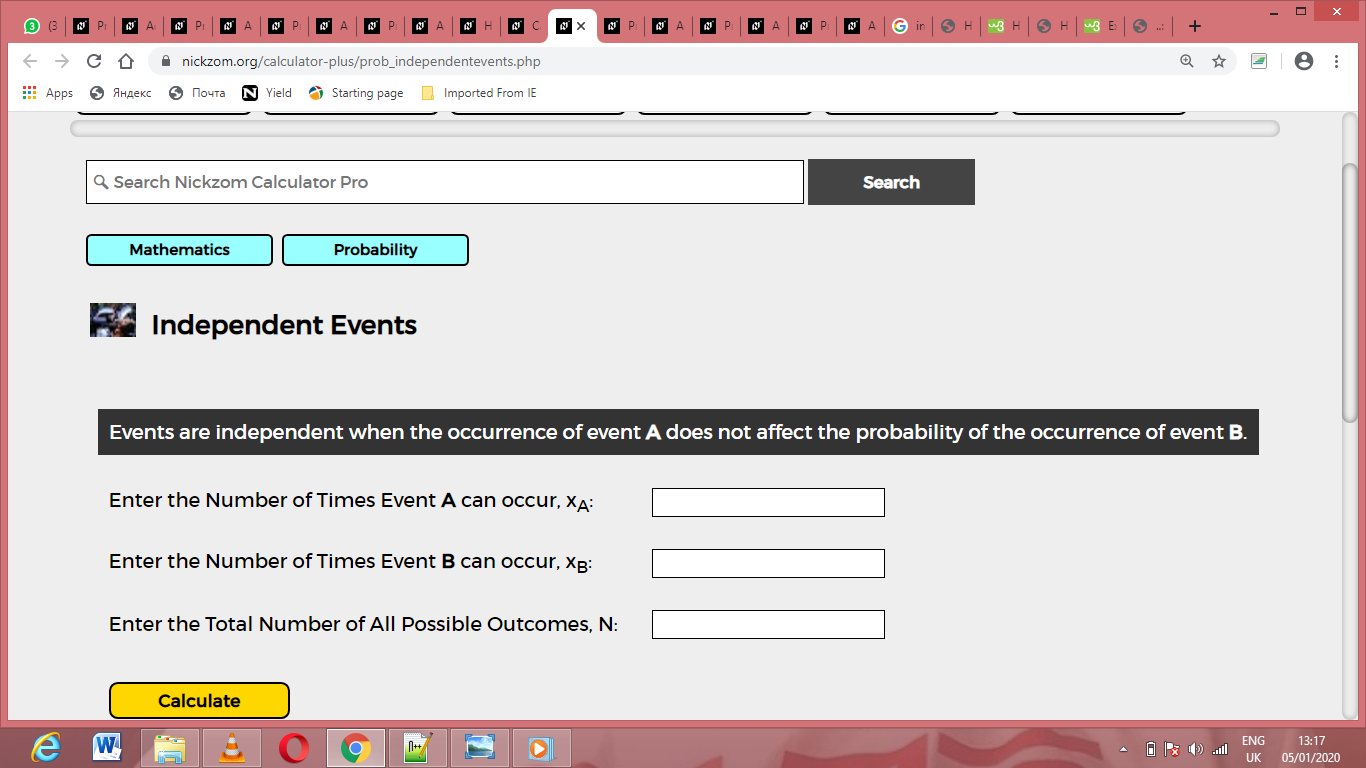Now, enter the values appropriately and accordingly for the parameters as required by the Number of Times Event A can occur(xA) is 11, Number of Times Event B can occur (xB) is 15 and Total Number of All Possible Outcomes(N) is 22.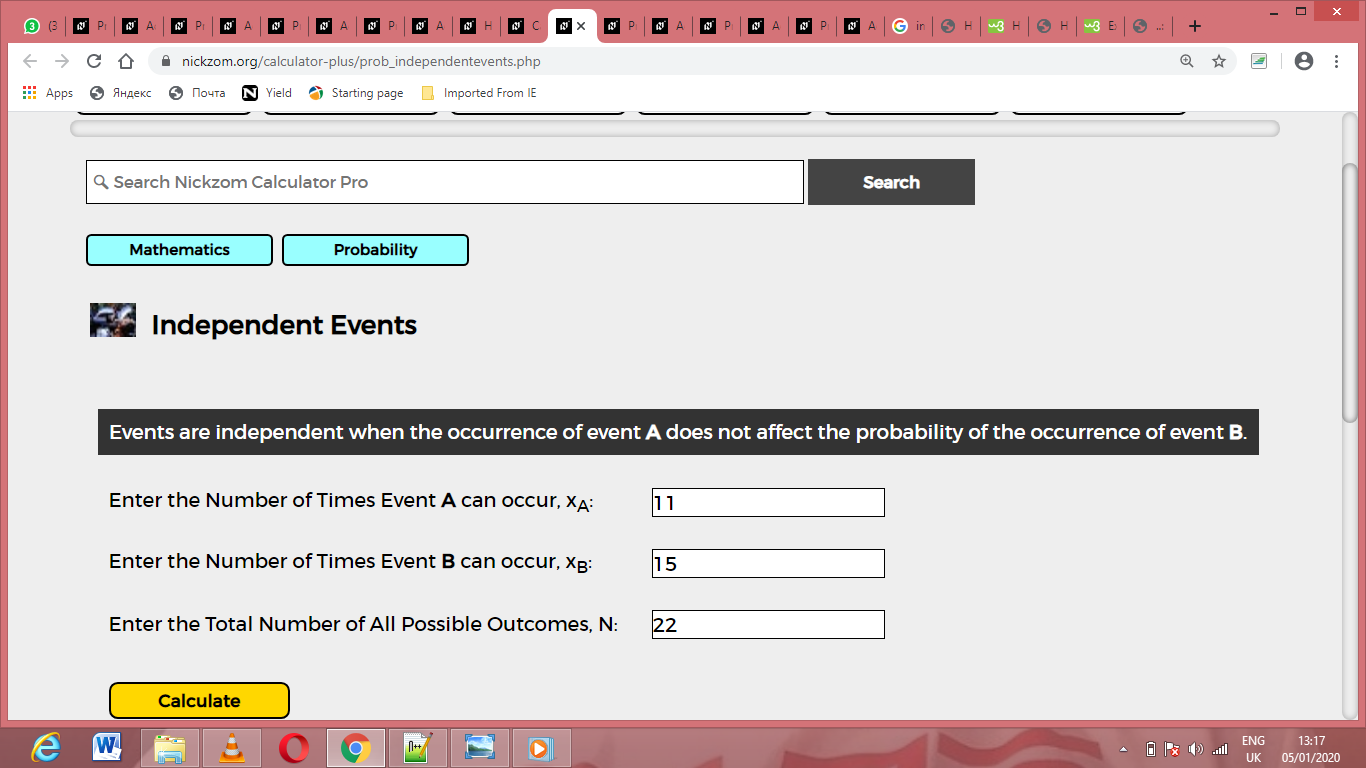Finally, Click on Calculate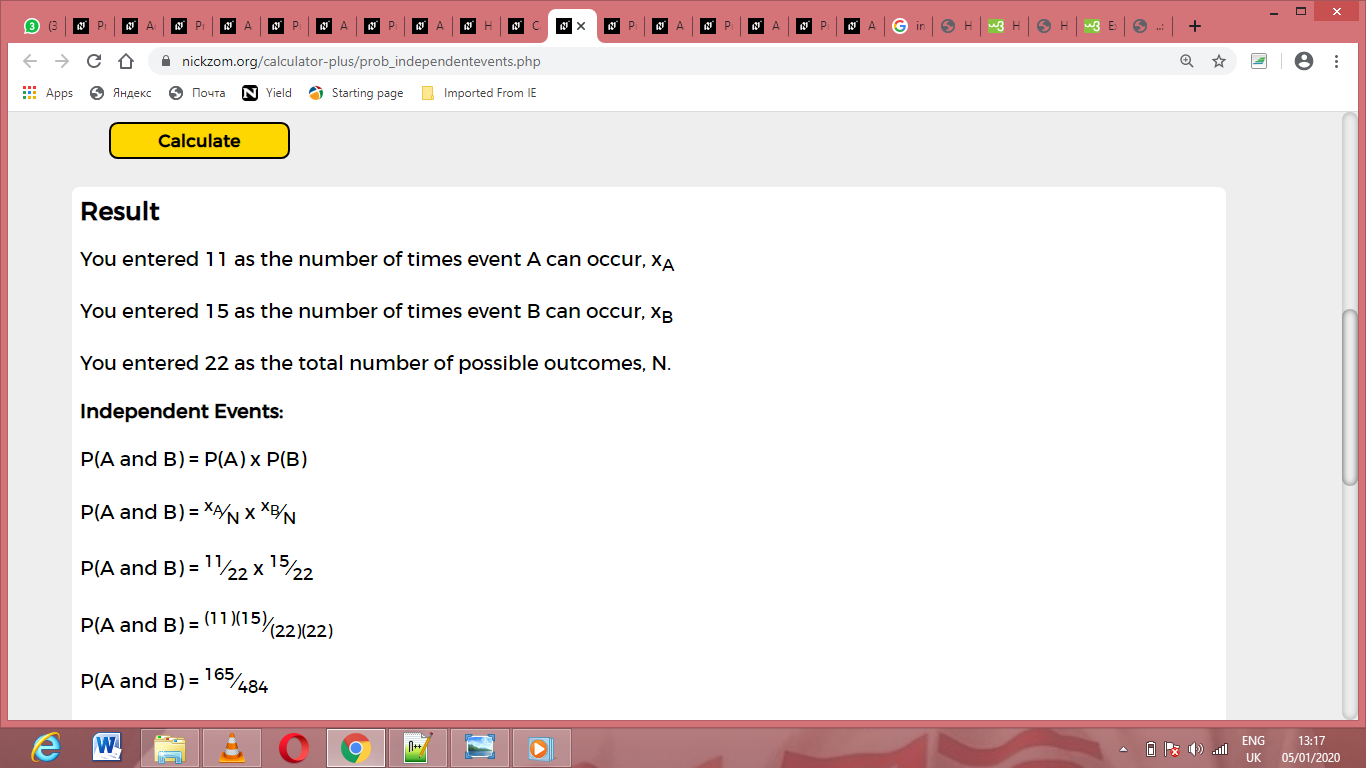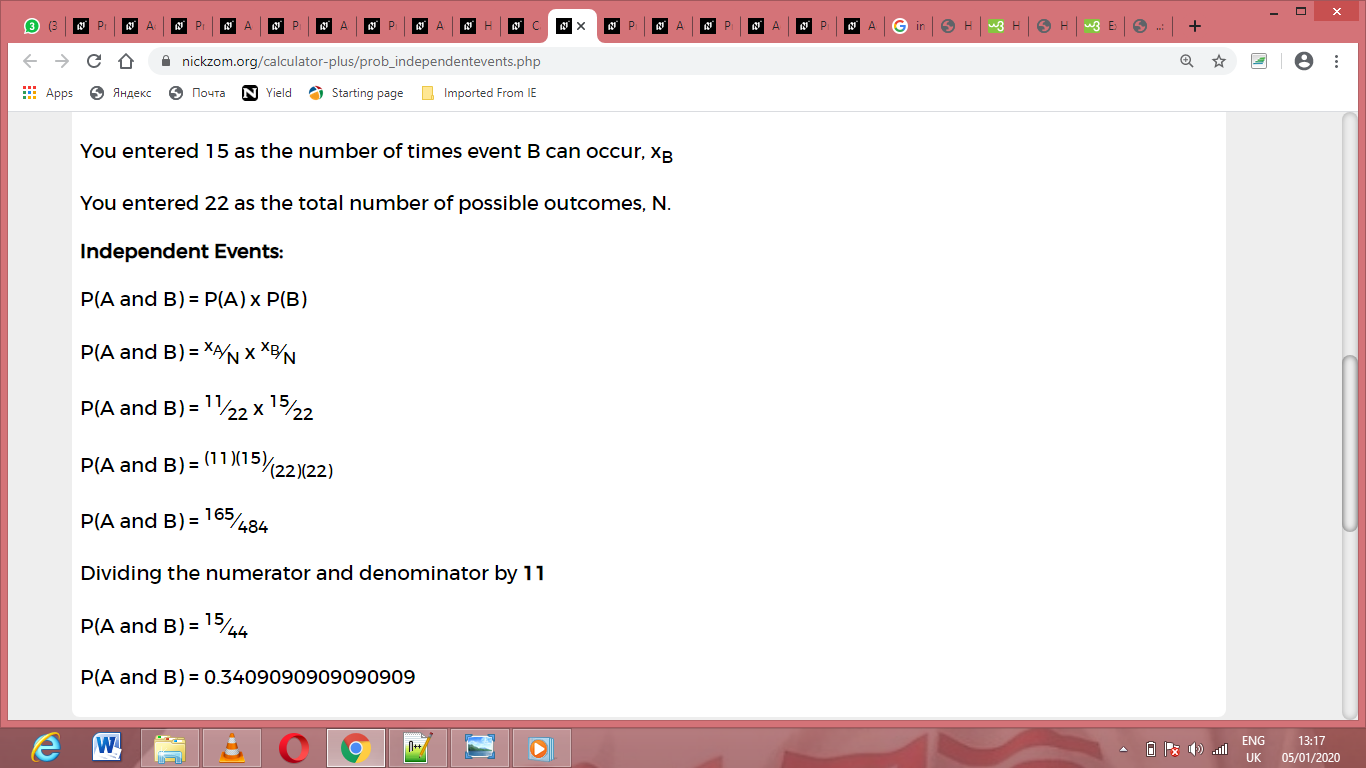As you can see from the screenshot above, Nickzom Calculator– The Calculator Encyclopedia solves for the independent events and presents the formula, workings and steps too.